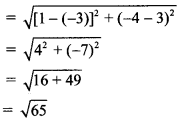Balbharti Maharashtra State Board Class 11 Maths Solutions Pdf Chapter 6 Circle Ex 6.1 Questions and Answers.

## Maharashtra State Board 11th Maths Solutions Chapter 6 Circle Ex 6.1

Question 1.
Find the equation of a circle with
(i) centre at origin and radius 4.
(ii) centre at (-3, -2) and radius 6.
(iii) centre at (2, -3) and radius 5.
(iv) centre at (-3, -3) passing through point (-3, -6).
Solution:
(i) The equation of a circle with centre at origin and radius ‘r’ is given by
x2 + y2 = r2
Here, r = 4
∴ The required equation of the circle is x2 + y2 = 42 i.e., x2 + y2 = 16.

(ii) The equation of a circle with centre at (h, k) and radius ‘r’ is given by
(x – h)2 + (y – k)2 = r2
Here, h = -3, k = -2 and r = 6
∴ The required equation of the circle is
[x – (-3)]2 + [y – (-2)]2 = 62
⇒ (x + 3)2 + (y + 2)2 = 36
⇒ x2 + 6x + 9 + y2 + 4y + 4 – 36 = 0
⇒ x2 + y2 + 6x + 4y – 23 = 0(iii) The equation of a circle with centre at (h, k) and radius ‘r’ is given by
(x – h)2 + (y – k)2 = r2
Here, h = 2, k = -3 and r = 5
The required equation of the circle is
(x – 2)2 + [y – (-3)]2 = 52
⇒ (x – 2)2 + (y + 3)2 = 25
⇒ x2 – 4x + 4 + y2 + 6y + 9 – 25 = 0
⇒ x2 + y2 – 4x + 6y – 12 = 0

(iv) Centre of the circle is C (-3, -3) and it passes through the point P (-3, -6).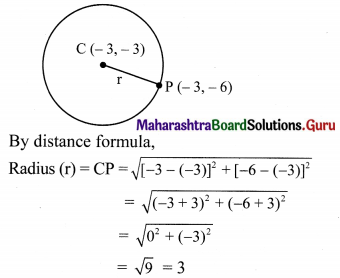The equation of a circle with centre at (h, k) and radius ‘r’ is given by
(x – h)2 + (y – k)2 = r2
Here, h = -3, k = -3, r = 3
The required equation of the circle is
[x – (-3)]2 + [y – (-3)]2 = 32
⇒ (x + 3)2 + (y + 3)2 = 9
⇒ x2 + 6x + 9 + y2 + 6y + 9 – 9 = 0
⇒ x2 + y2 + 6x + 6y + 9 = 0

Check:
If the point (-3, -6) satisfies x2 + y2 + 6x + 6y + 9 = 0, then our answer is correct.
L.H.S. = x2 + y2 + 6x + 6y + 9
= (-3)2 + (-6)2 + 6(-3) – 6(-6) + 9
= 9 + 36 – 18 – 36 + 9
= 0
= R.H.S.

Question 2.
Find the centre and radius of the following circles:
(i) x2 + y2 = 25
(ii) (x – 5)2 + (y – 3)2 = 20
(iii) $$\left(x-\frac{1}{2}\right)^{2}+\left(y+\frac{1}{3}\right)^{2}=\frac{1}{36}$$
Solution:
(i) Given equation of the circle is
x2 + y2 = 25
⇒ x2 + y2 = (5)2
Comparing this equation with x2 + y2 = r2, we get r = 5
Centre of the circle is (0, 0) and radius of the circle is 5.

(ii) Given equation of the circle is
(x – 5)2 + (y – 3)2 = 20
⇒ (x – 5)2 + (y – 3)2 = (√20)2
Comparing this equation with (x – h)2 + (y – k)2 = r2, we get
h = 5, k = 3 and r = √20 = 2√5
Centre of the circle = (h, k) = (5, 3)
and radius of the circle = 2√5.(iii) Given the equation of the circle is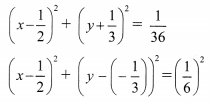Comparing this equation with (x – h)2 + (y – k)2 = r2, we get
h = $$\frac{1}{2}$$, k = $$\frac{-1}{3}$$ and r = $$\frac{1}{6}$$
Centre of the circle = (h, k) = ($$\frac{1}{2}$$, $$\frac{-1}{3}$$) and radius of the circle = $$\frac{1}{6}$$

Question 3.
Find the equation of the circle with centre
(i) at (a, b) and touching the Y-axis.
(ii) at (-2, 3) and touching the X-axis.
(iii) on the X-axis and passing through the origin having radius 4.
(iv) at (3, 1) and touching the line 8x – 15y + 25 = 0.
Solution:
(i) Since the circle is touching the Y-axis, the radius of the circle is X-co-ordinate of the centre.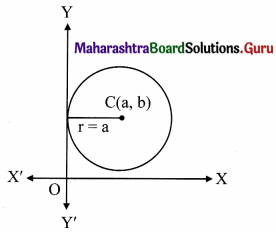∴ r = a
The equation of a circle with centre at (h, k) and radius r is given by
(x – h)2 + (y – k)2 = r2
Here, h = a, k = b
The required equation of the circle is
⇒ (x – a)2 + (y – b)2 = a2
⇒ x2 – 2ax + a2 + y2 – 2by + b2 = a2
⇒ x2 + y2 – 2ax – 2by + b2 = 0

(ii) Since the circle is touching the X-axis, the radius of the circle is the Y co-ordinate of the centre.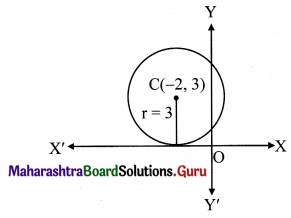∴ r = 3
The equation of a circle with centre at (h, k) and radius r is given by
(x – h)2 + (y – k)2 = r2
Here, h = -2, k = 3
The required equation of the circle is
⇒ (x + 2)2 + (y – 3)2 = 32
⇒ x2 + 4x + 4 + y2 – 6y + 9 = 9
⇒ x2 + y2 + 4x – 6y + 4 = 0(iii) Let the co-ordinates of the centre of the required circle be C (h, 0).
Since the circle passes through the origin i.e., O(0, 0)
⇒ $$\sqrt{(h-0)^{2}+(0-0)^{2}}=4$$
⇒ h2 = 16
⇒ h = ±4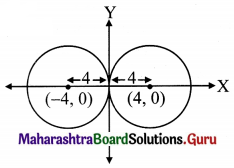the co-ordinates of the centre are (4, 0) or (-4, 0).
The equation of a circle with centre at (h, k) and radius r is given by
(x – h)2 + (y – k)2 = r2
Here, h = ± 4, k = 0, r = 4
The required equation of the circle is
⇒ (x – 4)2 + (y – 0)2 = 42 or (x + 4)2 + (y – 0)2 = 42
⇒ x2 – 8x + 16 + y2 = 16 or x2 + 8x + 16 + y2 = 16
⇒ x2 + y2 – 8x = 0 or x2 + y2 + 8x = 0

(iv) Centre of the circle is C (3, 1).
Let the circle touch the line 8x – 15y + 25 = 0 at point M.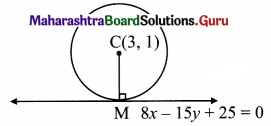CM = Length of perpendicular from centre C(3, 1) on the line 8x – 15y + 25 = 0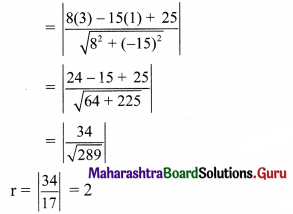The equation of a circle with centre at (h, k) and radius r is given by
(x – h)2 + (y – k)2 = r2
Here, h = 3, k = 1 and r = 2
The required equation of the circle is
⇒ (x – 3)2 + (y – 1)2 = 22
⇒ x2 – 6x + 9 + y2 – 2y + 1 = 4
⇒ x2 + y2 – 6x – 2y + 10 – 4 = 0
⇒ x2 + y2 – 6x – 2y + 6 = 0

Question 4.
Find the equation of the circle, if the equations of two diameters are 2x + y = 6 and 3x + 2y = 4 and radius is 9.
Solution: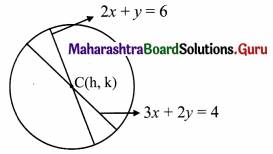Given equations of diameters are 2x + y = 6 and 3x + 2y = 4.
Let C (h, k) be the centre of the required circle.
Since point of intersection of diameters is the centre of the circle,
x = h, y = k
Equations of diameters become
2h + k = 6 …..(i)
and 3h + 2k = 4 ……..(ii)
By (ii) – 2 × (i), we get
-h = -8
⇒ h = 8
Substituting h = 8 in (i), we get
2(8) + k = 6
⇒ k = 6 – 16
⇒ k = -10
Centre of the circle is C (8, -10) and radius, r = 9
The equation of a circle with centre at (h, k) and radius r is given by
(x – h)2 + (y – k)2 = r2
Here, h = 8, k = -10
The required equation of the circle is
⇒ (x – 8)2 + (y + 10)2 = 92
⇒ x2 – 16x + 64 + y2 + 20y + 100 = 81
⇒ x2 + y2 – 16x + 20y + 100 + 64 – 81 = 0
⇒ x2 + y2 – 16x + 20y + 83 = 0Question 5.
If y = 2x is a chord of the circle x2 + y2 – 10x = 0, find the equation of the circle with this chord as diameter.
Solution: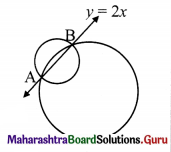y = 2x is the chord of the given circle.
It satisfies the equation of a given circle.
Substituting y = 2x in x2 + y2 – 10x = 0, we get
⇒ x2 + (2x)2 – 10x = 0
⇒ x2 + 4x2 – 10x = 0
⇒ 5x2 – 10x = 0
⇒ 5x(x – 2) = 0
⇒ x = 0 or x = 2
When x = 0, y = 2x = 2(0) = 0
∴ A = (0, 0)
When x = 2, y = 2x = 2 (2) = 4
∴ B = (2, 4)
End points of chord AB are A(0, 0) and B(2, 4).
Chord AB is the diameter of the required circle.
The equation of a circle having (x1, y1) and (x2, y2) as end points of diameter is given by
(x – x1) (x – x2) + (y – y1) (y – y2) = 0
Here, x1 = 0, y1 = 0, x2 = 2, y2 = 4
The required equation of the circle is
⇒ (x – 0) (x – 2) + (y – 0) (y – 4 ) = 0
⇒ x2 – 2x + y2 – 4y = 0
⇒ x2 + y2 – 2x – 4y = 0

Question 6.
Find the equation of a circle with a radius of 4 units and touch both the co-ordinate axes having centre in the third quadrant. Solution:
The radius of the circle = 4 units
Since the circle touches both the co-ordinate axes and its centre is in the third quadrant,
the centre of the circle is C(-4, -4).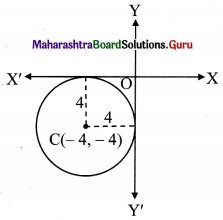The equation of a circle with centre at (h, k) and radius r is given by (x – h)2 + (y – k)2 = r2
Here, h = -4, k = -4, r = 4
the required equation of the circle is
⇒ [x – (-4)]2 + [y – (-4)]2 = 42
⇒ (x + 4)2 + (y + 4)2 = 16
⇒ x2 + 8x + 16 + y2 + 8y + 16 – 16 = 0
⇒ x2 + y2 + 8x + 8y + 16 = 0Question 7.
Find the equation of the circle passing through the origin and having intercepts 4 and -5 on the co-ordinate axes.
Solution:
Let the circle intersect X-axis at point A and intersect Y-axis at point B.
the co-ordinates of point A are (4, 0) and the co-ordinates of point B are (0, -5).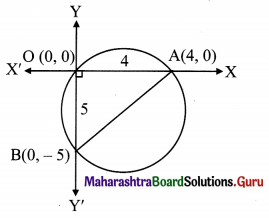Since ∠AOB is a right angle,
AB represents the diameter of the circle.
The equation of a circle having (x1, y1) and (x2, y2) as end points of diameter is given by
(x – x1) (x – x2) + (y – y1) (y – y2) = 0
Here, x1 = 4, y1 = 0, x2 = 0, y2 = -5
The required equation of the circle is
⇒ (x – 4) (x – 0) + (y – 0) [y – (-5)] = 0
⇒ x(x – 4) + y(y + 5) = 0
⇒ x2 – 4x + y2 + 5y = 0
⇒ x2 + y2 – 4x + 5y = 0

Question 8.
Find the equation of a circle passing through the points (1, -4), (5, 2) and having its centre on line x – 2y + 9 = 0.
Solution: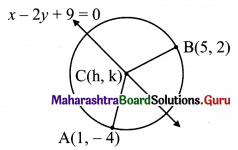Let C(h, k) be the centre of the required circle which lies on the line x – 2y + 9 = 0.
Equation of line becomes
h – 2k + 9 = 0 …..(i)
Also, the required circle passes through points A(1, -4) and B(5, 2).
CA = CB
By distance formula,
$$\sqrt{(\mathrm{h}-1)^{2}+[\mathrm{k}-(-4)]^{2}}=\sqrt{(\mathrm{h}-5)^{2}+(\mathrm{k}-2)^{2}}$$
Squaring both the sides, we get
⇒ (h – 1)2 + (k + 4)2 = (h – 5)2 + (k – 2)2
⇒ h2 – 2h + 1 + k2 + 8k + 16 = h2 – 10h + 25 + k2 – 4k + 4
⇒ -2h + 8k + 17 = -10h – 4k + 29
⇒ 8h + 12k – 12 = 0
⇒ 2h + 3k – 3 = 0 ……(ii)
By (ii) – (i) × 2, we get
7k = 21
⇒ k = 3
Substituting k = 3 in (i), we get
h – 2(3) + 9 = 0
⇒ h – 6 + 9 = 0
⇒ h = -3
Centre of the circle is C(-3, 3).